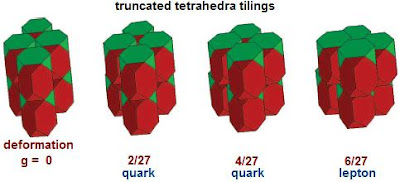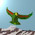## Thursday, January 12, 2012

### Theory Update 135

A fascinating new paper by Yang Jiao and Salvatore Torquato defines three dimensional packings of truncated tetrahedra, using a lattice vector deformation parameter $g$ that takes values in the interval $[0,2/9]$.A trucated tetrahedron has four hexagonal faces and four triangular ones. The maximal $g = 2/9$ corresponds to the densest known packing of truncated tetrahedra, with packing fraction $207/208$. The packing has tetrahedra shaped holes. With Conway they have also constructed a packing with fraction $23/24$, and considered packings involving octahedra.

#### 4 comments:

1.Kea,

One of my favorite space fillers as a simi-regular tesselation (for storage containers and with 1/4 of a tetrahedron added.

I like your link to Conway involving octahedra although many many concave structures are possible.

These shapes, even the polytopes, should be seen from a higher dimensional view rather than that hidden by our usual 3d traditions and classifications. The complexity of shapes so reduced hide the deep significance and in a way when we have simplified algebra, things done in relation to one dimensional curves in a plane- the deep significance is also hidden. I do not know which is easier or how much of that now known in mathematics still hidden from my view in the exquisite detail of the beauty.

In a wider sense, in the structure that underlies planes... the tetrahedron is a space filler if we consider all permutations and dimensions- by this I do not simply mean the 4 triangle solid nor its group coordinates.

The PeSla Some still think this way to view symmetry is not that important.

2.Very interesting. Of course the 0/27 corresponds to the neutrinos and what you've labeled as "lepton" actually is the charged leptons only.

The tetrahedron suggests a space with four directions. Does this have to do with the four mutually unbiased bases available in 3-dimensional Hilbert space? Does that mean that the 3-dimensions of the above illustrations are the three quark colors?

A very interesting post.

3.The truncated tetrahedron paper is indeed interesting.
For example:

only the gamma = 0 and gamma = 2/9 values correspond to tilings
while
the open interior (0 , 2/9 ) which includes 2/27 and 4/27 for quarks dpes not correspond to any tiling

for gamma = 2/9 "... each dimer has 14 face-to-face contacts ..."
Do those 14 contacts form the vertices of a rhombic dodecahedron?

for gamma = 0 it appears that each dimer has 6 face-to-face contacts
Do those 6 contacts form the vertices of an octahedron?

If the answer to those 2 questions is "Yes"
then
does the transformation of sliding dimers from gamma = 0 to gamma = 2/9
correspond to
a Fuller Jitterbug-type transformation from octahedron to rhombic dodecahedron?

If so, since the standard Fuller Jitterbug goes from octahedron to cuboctahedron with an intermediate icosahedron,
is there an intermediate dodecahedron (with no tiling) state between gamma = 0 (octahedron) and gamma = 2/9 (rhombic dodecahedron) ?

Tony

4.This post has nothing at all to do with my favourite ideas for tackling the $2/9$ etc, as The PeSla has noted.

Note: Only a member of this blog may post a comment.